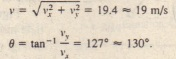# Sample Problem Motion In Two Three Dimensions

Sample Problem Motion In Two Three Dimensions

A particle velocity (in meters per second) at 1 = 0 undergoes a constant acceleration a of magnitude from the positive direction of the x axis. What is the particle’s velocity , in unit vector notation and as a magnitude and an angle?

SOLUTION: We first note that this is two-dimensional motion, in the A) plane. Then there are two Key Ideas here. One is that, because the acceleration is constant,  applies. The second is that, because  applies only to straight-line motion, we must apply it separately for motion parallel to the x axis and motion  parallel to the y axis; that is, we must find the velocity components from the equationsEither using a vector-capable calculator or following.We find that the magnitude and angle of V areCheck the last line with your calculator. Does appear on the display, or does 53° appear? Now sketch the vector V with its components to see which angle is reasonable.

CHECKPOINT:If the position of a hobo’s marble is given by with r in meters and t in seconds, what must be the units of the coefficients .